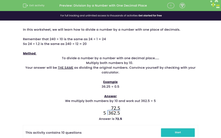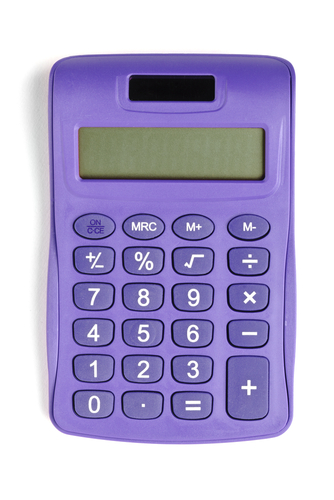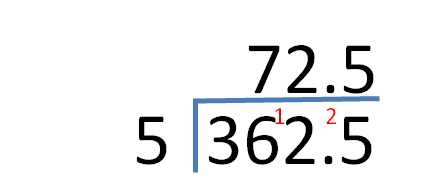# Divide by a Number with One Decimal Place

In this worksheet, students will divide by numbers with one decimal place.Key stage:  KS 3

Curriculum topic:   Number

Curriculum subtopic:   Use Four Operations for All Numbers

Difficulty level:#### Worksheet Overview

In this activity, we will learn how to divide a number by another number with one decimal place.

Remember that 240 ÷ 10 is the same as 24 ÷ 1 = 24

So 24 ÷ 1.2 is the same as 240 ÷ 12 = 20

Method

To divide a number by a number with one decimal place:

First of all, we need to multiply both numbers by 10.

You need to do the same thing to both numbers or it won't be correct!

Your answer will be the same as dividing the original numbers. Prove it to yourself by checking with your calculator!Example

36.25 ÷ 0.5

We multiply both numbers by 10 and work out 362.5 ÷ 5Does that make sense? It's simple really once you get a bit of practice in!

Let's get started!### What is EdPlace?

We're your National Curriculum aligned online education content provider helping each child succeed in English, maths and science from year 1 to GCSE. With an EdPlace account you’ll be able to track and measure progress, helping each child achieve their best. We build confidence and attainment by personalising each child’s learning at a level that suits them.

Get started